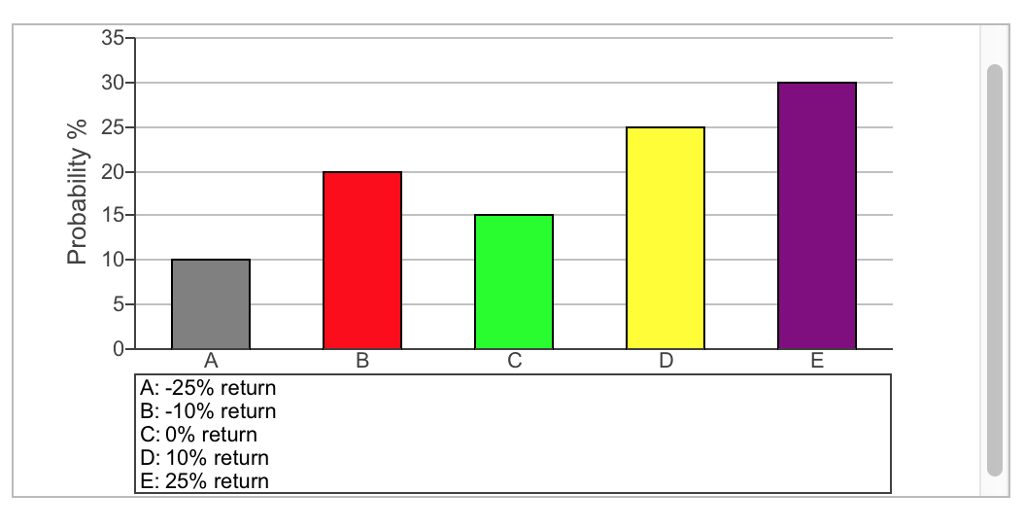### Create an Account

Home / Questions / Hi I need help with part b The figure in the popup window shown below shows the one-year ...

# Hi I need help with part b The figure in the popup window shown below shows the one-year return distribution for RCS stock Calculate a The expected return DONE b The standard

Hi, I need help with part b,:

The figure in the popup? window (shown below) shows the? one-year return distribution for RCS stock.?

Calculate:

a. The expected return. DONE

b. The standard deviation of the return. NEED HELP HERE

Note?: Make sure to round all intermediate calculations to at least five decimal places.

a. The expected return.

The expected return is

5.5%. CORRECT

b. The standard deviation of the return.

The standard deviation is ??%.30 A:-25% return B:-10% return C: 0% return D: 10% return E: 25% return E D C B nn uu A% % %% A-0/0 e r r 50%05 21 -012 ABCDE 50505050 332211 % All-igeqo-d

Apr 04 2020 View more View LessSubscribe To Get Solution